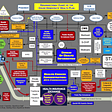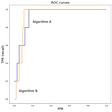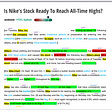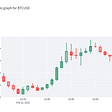# FX Volatility Surface Construction using the Vanna Volga approach with Python

Options as a product type form an important segment of the derivatives asset class. Options are used extensively by market participants including banks, financial institutions, insurance firms etc. be it for Trading or for Risk Management purposes.

A brief on basics of Options

For the benefit of readers, I will explain the basics of Options in a nutshell. Options are a type of a derivative which give the right but not the obligation to the holder/buyer of the Option to buy/sell a certain item at a pre-specified price and at a certain time in the future. The holder gets this right by paying a premium amount to the seller/writer of the Option. Option being a derivative product, derives its value from an underlying asset. The underlying asset for the options could be anything including FX, rates, equity, commodity etc. to name a few. Options get traded on both the regulated Exchanges (F&O segment that many of us know) as well as in the Over the Counter (OTC) market.

Why is pricing of options so important?!

Options form a large part of the portfolio of banks, financial institutions and other market participants. Options are very sensitive to a certain set of market risk factors (i.e. market inputs) which we will discuss in the next section. Movements in the aforesaid risk factors have an impact on the way options prices fluctuate. Option pricing is important as it impacts a host of other items. A few are included in the list below:

a. Capital Charge

c. Value-at-Risk

d. Collateral exchange

e. Exposure monitoring etc.

All of the above items are key elements from a risk management perspective. Incorrect option pricing can lead to erroneous computations of any/all of the above items. This is extremely detrimental for a bank/financial institution, as this will not only result in a non-optimized capital allocation, but will also attract harsh penalty from the Regulators. Therefore, market participants use sophisticated models for options pricing. The focus of these models is to arrive at a correct estimate of how much the option is worth today (this is also what we call as the MTM). Further, market participants are always on the lookout for potential enhancements that could be applied to further refine their existing option pricing framework.

Option Pricing:

The following set of risk factors are required to price an option contract:

a. Spot Price

b. Strike Price

c. Risk free rate

d. Time to Maturity

e. Volatility

From the above list, the first four risk factors are easy to figure out. There is no uncertainty/estimation needed for them. However, the final risk factor i.e. Volatility is a factor that needs to be estimated. From the above list of factors, volatility has a large bearing on an option’s price. There are popular models like Black Scholes Merton (BSM) model that are used to arrive at a volatility number (we also call this as the implied volatility). BSM has been around for a long time, and gives a descent estimate of plain vanilla option prices. However, BSM model is based on an assumption that volatility remains constant. This is a major shortcoming of the BSM model, the reason being, option volatilities are anything but constant. Therefore, market participants have been using alternatives to the BSM model i.e. stochastic volatility models or models like Vanna Volga (also referred to as “VV” hereinafter) etc. to overcome the constant volatility assumption used in BSM.

Stochastic volatility models are very sophisticated and elaborate when it comes to volatility modelling and give good estimates of volatility. Stochastic models are highly quantitative in nature and it takes considerable development effort for model implementation. We will explore stochastic volatility models in a future post

In this post we will explore the Vanna Volga approach for FX Vols surface construction. We will discuss the basic theoretical underpinnings of VV approach and a Python implementation of the same in order to construct an FX vols surface. There is a significant amount of quantitative rigor for VV approach, however, in this post I have not included the complex mathematical equations that go into this approach (although the implementation of the same has been done in the Python code). In the references section, I have included the details of a research paper on Vanna Volga approach which explains the underlying quantitative aspects of this model for interested readers to go through further to reading this article.

Vanna Volga Approach to FX Vols surface construction:

FX Options are one of the most widely traded financial contracts in the market. In FX markets, three main volatility quotes i.e. the most liquid volatility quotes available i.e. ATM, 25 Delta Call, 25 Delta Put. In this article we will discuss the usage of VV approach to derive an implied volatility for all options’ delta (i.e. we will build an entire volatility surface) which we can then use for pricing options other than the three aforesaid ones. Our aim is to have a smile consistent volatility for accurate pricing of options. VV approach involves an adjustment over the Black Scholes model. In VV, we account for the volatility smile adjustment i.e. we adjust the BSM value by the cost of a portfolio which hedges the three main sources of risk related to volatility namely, Vega (Rate of change of option price w.r.t. change in implied volatility), Vanna (Rate of change of Vega w.r.t underlying spot price) and Volga (Rate of change of Vega w.r.t implied volatility). For this purpose, we use the two most liquid strategies in the FX markets i.e. the Risk Reversal (RR) and Butterfly (BF). We attempt to capture the quantity Vanna via the RR, and the quantity Volga via the BF. The adjustment is given as below:

VV = BS + [(wRR) X RRcost] + [(wBF) X (BFcost)]

Where, BS is the Black scholes price,

VV is the Vanna Volga price

wRR: the quantity of RR needed to replicate the Vanna

wBF: the quantity of BF needed to replicate the Volga

The logic implemented in the above formula (i.e. difference between VV price and BS price given be VV — BS) is to incorporate the smile cost of hedging the vega, vanna and volga risks.

The above weights can be computed by solving a set of equations.

The advantage of VV approach is that the weights derived have a closed form solution. This permits an explicit construction of the volatility smile. VV technique satisfies the required no-arbitrage conditions, and when by changing the three initial pairs of strikes and volatility will produce a consistent implied vol curve. Under the technique of vol surface construction, we also solve for first and second order approximation of implied volatility induced by the VV model price. VV thus, provides an efficient and a fast way to interpolate and extrapolate implied volatilities. These can be subsequently used for pricing various options.

Below, is the Python implementation for FX vols surface construction:

1. Import the required libraries in the program:

import numpy as np
import pandas as pd
import matplotlib.pyplot as plt
import scipy.stats as st
from mpl_toolkits import mplot3d
import math
import matplotlib as mpt

2. Define the main function which defines the starting point of the program flow:

if __name__==’__main__’:
main()

3. Input hypothetical market data points for the following:

Time tenors, 25D Put, 25D Call and ATM, interest rates in both currencies

#Time tenor points
T = np.array([0.0194,0.04166,0.0833,0.1666,0.25,0.3333,0.4166,0.5,0.75,1,1.25,1.5,2,3,4,5])

#Hypothetical volatility quotes for three most liquid points on the vol surface 25D Put, 25D Call and ATM
Vol_25D_PUT = np.array([0.121,0.1215,0.1105,0.113,0.1224,0.1236,0.125,0.116,0.1175,0.1322,0.136,0.14,0.1411,0.1433,0.1445,0.145])
Vol_25D_CALL = np.array([0.1205,0.12,0.115,0.109,0.1125,0.121,0.119,0.108,0.116,0.1275,0.131,0.133,0.1388,0.14,0.1405,0.139])
Vol_ATM = np.array([0.118,0.1182,0.1015,0.1029,0.115,0.116,0.118,0.105,0.108,0.121,0.124,0.132,0.135,0.1375,0.14,0.141])

#Hypothetical interest rates in two currencies
rd_input = np.array([0.005,0.0052,0.0059,0.006,0.0063,0.0069,0.007,0.0072,0.0075,0.0077,0.008,0.0085,0.009,0.00925,0.0095,0.0098])
rf_input = np.array([0.0043,0.004,0.005,0.0055,0.0068,0.0071,0.0066,0.0078,0.0085,0.0083,0.0088,0.0079,0.0082,0.0087,0.0093,0.0095])

4. Calculation of Discount factors needed in the computation:

#Calculate Discount factors
rd_discFact = np.exp(-1 * np.multiply(rd_input,T))
rf_discFact = np.exp(-1 * np.multiply(rf_input,T))

q = rd_discFact-rf_discFact #rd — rf

5. Create empty arrays, forwards etc.

#creating empty arrays to hold X (strikes)
X_3 = np.array([])
X_1 = np.array([])
X_2 = np.array([])

S = 1.5
delta = 0.25
F = S * np.exp(np.multiply(q,T))
a = -1 * st.norm.ppf(delta * (1/rf_discFact))

6. Calculate d1 and d2 for the option pricing equation:

# d1 part of option pricing formula
def d_1(F,X,vol,t):
d1 = (math.log(F/X) + 0.5 * (vol **2) * t)/(vol * math.sqrt(t))
return d1

# d2 part of the option pricing formula
def d_2(F,X,vol,t):
d2 = d_1(F,X,vol,t) — vol * math.sqrt(t)
return d2

7. Calculation for strikes

for x in range(0,len(T)):

X_25D_PUT = F[x] * math.exp(-(a[x]*Vol_25D_PUT[x]*math.sqrt(T[x]))+0.5 * (Vol_25D_PUT[x]**2)*T[x])
X_1 = np.append(X_1,X_25D_PUT)

X_ATM = F[x] * math.exp(0.5 * (Vol_ATM[x] ** 2) * T[x])
X_2 = np.append(X_2, X_ATM)

X_25D_CALL = F[x] * math.exp((a[x]*Vol_25D_CALL[x]*math.sqrt(T[x]))+ 0.5 * (Vol_25D_CALL[x]**2)*T[x])
X_3 = np.append(X_3,X_25D_CALL)

8. Weights and first and second order partial derivatives

#weights and srikes
def VolSurface(F,X,t,X_1,X_2,X_3,sig_PUT,sig_ATM,sig_CALL):
z1 = (math.log(X_2/X) * math.log(X_3/X))/(math.log(X_2/X_1) * math.log(X_3/X_1))
z2 = (math.log(X/X_1) * math.log(X_3/X))/(math.log(X_2/X_1) * math.log(X_3/X_2))
z3 = (math.log(X/X_1) * math.log(X/X_2))/(math.log(X_3/X_1) * math.log(X_3/X_2))

First_Ord_Approx = (z1 * sig_PUT + z2 * sig_ATM + z3 * sig_CALL) — sig_ATM
Second_Ord_Approx = z1 * d_1(F,X_1,sig_PUT,t) * d_2(F,X_1,sig_PUT,t) * ((sig_PUT-sig_ATM)**2) \
+z2 * d_1(F,X_2,sig_ATM,t) * d_2(F,X_2,X_ATM,t) * ((sig_ATM-sig_ATM)**2) \
+ z3 * d_1(F,X_3,sig_CALL,t) * d_2(F,X_3,sig_CALL,t) * ((sig_CALL-sig_ATM)**2)

d1_d2 = d_1(F,X,sig_ATM,t) * d_2(F,X,sig_ATM,t)

vol = sig_ATM + (-sig_ATM + math.sqrt(sig_ATM**2 + d1_d2 * (2 * sig_ATM * First_Ord_Approx+Second_Ord_Approx)))/(d1_d2)

return vol

`opt_strike = (1 + np.arange(-0.15,0.16,0.01))* Svanna_volga_implied = np.zeros((31,16),dtype=float)`

9. Construct vol surface and save in dataframe

#building the vol surface
for i in range(31):
for j in range(16):
vanna_volga_implied[i][j] = VolSurface(F[j],opt_strike[i],T[j],X_1[j],X_2[j],X_3[j],Vol_25D_PUT[j],Vol_ATM[j],Vol_25D_CALL[j])

df = pd.DataFrame(vanna_volga_implied)
df.to_csv(‘e:\\ameya.csv’,index=False)

10. Plot Vol surface using matplotlib

#Plotting the vol surface using matplotlib
x_axis = T
y_axis = opt_strike
z_axis = df
x_axis,y_axis = np.meshgrid(x_axis,y_axis)
fig = plt.figure()
vol_surface = ax.plot_surface(x_axis,y_axis,z_axis,linewidth = 0,antialiased=False)
plt.show(True)

The main advantage of VV is the speed with we can generate implied volatilities. This makes the model very appealing to traders for making trading decisions, also VV is useful to risk managers for the purpose of pricing and risk management of options portfolios.

In this article we have used one approach to build an FX volatility surface using powerful libraries in Python. Further, it would be worth exploring other sophisticated machine learning libraries which Python offers in order to see if the surface can be constructed in another way which may be more efficient and faster as well.

References:

“The Vanna Volga method for implied volatilities”, Risk magazine — by Castagna and Mercurio

****

--

--

--

## More from Ameya Abhyankar

Founder: FinQuest Institute | Ekspert Consulting; www.finquestinstitute.com; www.ekspertconsulting.com

Love podcasts or audiobooks? Learn on the go with our new app.

## Can you trust your model when data shifts?## DS4A Capstone Project Spotlight — Farmacy?:## How to generate dummy data in Python## The Power of Art in Politics:## Decolonising Data## Deploy Keras Models locally using TensorFlow Serving — TF 2.x## PM 101: Pitfalls of A/B Testing## Evaluating anomaly detection algorithms with receiver operating characteristic (ROC) curves## Ameya Abhyankar

Founder: FinQuest Institute | Ekspert Consulting; www.finquestinstitute.com; www.ekspertconsulting.com

## How to backtest Quantconnect’s (LEAN) strategies locally using Yahoo data and Python## Selling the Hype: Coding Sentiment Analysis for Stock Market News in 4 STEPS## Introduction to backtesting strategy: Historical data replay in DolphinDB## Financial Data Streaming using Alpaca and Streamlit